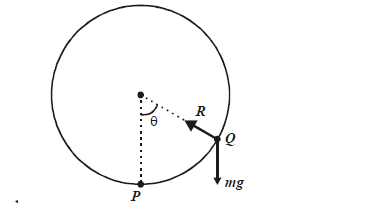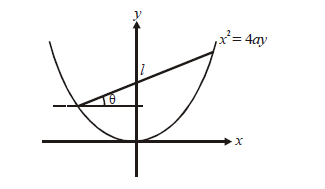# Applications of Derivatives Set 13

Go back to  'SOLVED EXAMPLES'

Example - 29

Consider a particle free to move on the inner side of a smooth vertical circular ring of radius r. It is projected from the lowest point with a velocity just sufficient to carry it to the highest point. Show that  the reaction between the particle and the ring is zero after time t given by

$t = \sqrt {\frac{r}{g}} \;\log \left( {\sqrt 5 + \sqrt 6 } \right)$

Solution:         Let P be the lowest point and Q be the point at which the reaction becomes zero. Let $${v_i}$$and $${v_f}$$ be the respective velocities of the particle t = 0 and later instant.By energy conservation, we have

$\frac{1}{2}mv_i^2 - \frac{1}{2}mv_f^2 = mgr(1 - \cos \theta )$

At $$\theta = \pi ,\;{v_f} = 0$$

$\Rightarrow \frac{1}{2}mv_i^2 = 2\;mgr$

At the point    $$Q,\;v_f^2 = 2gr(1 + \cos {\theta _Q})$$

$R = 0 = \frac{{mv_{f\theta }^2}}{r} + mg\cos {\theta _Q} \Rightarrow \cos {\theta _Q} = - \frac{2}{3}$

Now,

\begin{align}&{v_f} = \frac{{rd\theta }}{{dt}} = \sqrt {2gr(1 + \cos \theta )} = 2\sqrt {gr} \cos \frac{\theta }{2}\\\\ &\Rightarrow \quad dt = \frac{1}{2}\sqrt {\frac{r}{g}} \sec \frac{\theta }{2}d\theta \Rightarrow {t_Q} = \frac{1}{2}\sqrt {\frac{r}{g}} \;\int\limits_0^{{\theta _Q}} {\sec \frac{\theta }{2}\;d\theta } \\\\&\,\,\,\,\,\,\,\,\,\,\,\,\,\,\,\,\,\,\,\, = \sqrt {\frac{r}{g}} \log \;\sec \frac{{{\theta _Q}}}{2} + \tan \frac{{{\theta _Q}}}{2}\\\\&\,\,\,\,\,\,\,\,\,\,\,\,\,\,\,\,\,\, = \sqrt {\frac{r}{g}} \log \left( {\sqrt 6 + \sqrt 5 } \right)\end{align}

Example - 30

Consider the parabola $${x^2} = 4ay,\;a > 0$$. A rod of length l (greater than the semi-latus-rectum of the parabola) rests on the parabola as shown.Let $$\theta$$ be the inclination of the rod. Find the value(s) of  $$\theta$$ for which the height of the center of the rod is minimum.

Solution: From the geometry of the figure,

$P \equiv \left( {2at,\;a{t^2}} \right),\;\theta \equiv \left( {2at + 2l\cos \theta ,\;a{t^2} + 2l\sin \theta } \right)$

From the fact that Q must satisfy $${x^2}4ay,$$we obtain

$t = \tan \theta - \frac{l}{{2a}}\cos \theta$

Therefore, the height is

$h = a\,{t^2} + l\sin \theta = a{\tan ^2}\theta + \frac{{{l^2}}}{{4{a^2}}}{\cos ^2}\theta$

$$h(\theta )$$has extreme (minima) points for \begin{align}{\cos ^2}\theta = \frac{{2a}}{l}\;{\rm{or}}\;\sin \theta = 0\end{align}

Learn from the best math teachers and top your exams

• Live one on one classroom and doubt clearing
• Practice worksheets in and after class for conceptual clarity
• Personalized curriculum to keep up with school